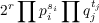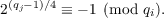#### Vol. 60, No. 1, 1975

 Download this articleFor screen For printingRecent Issues Vol. 325: 1  2 Vol. 324: 1  2 Vol. 323: 1  2 Vol. 322: 1  2 Vol. 321: 1  2 Vol. 320: 1  2 Vol. 319: 1  2 Vol. 318: 1  2Online Archive Volume: Issue:The Journal Subscriptions Editorial Board Officers Contacts Submission Guidelines Submission Form Policies for Authors ISSN: 1945-5844 (e-only) ISSN: 0030-8730 (print) Special Issues Author Index To Appear Other MSP Journals
The Diophantine equation Y (Y + m)(Y + 2m)(Y + 3m) = 2X(X + m)(X + 2m)(X + 3m)

### S. Jeyaratnam

Vol. 60 (1975), No. 1, 183–187
##### Abstract

J. H. E. Cohn has shown that the equation of the tltle has only four pairs of nontrivial solutions in integers when m = 1. The object of this paper is to prove the following two theorems concerning the solutions of the equation of the title:

Theorem 1. The equation of the title has only four pairs of nontrivial solutions in integers given by X = 4m or 7m, Y = 5m or 8m when m is of the formwhere r, si’s and tj’s are nonnegative integers, pi’s are positive primes 3,5 (mod 8) and qi’s are positive primes 1 (mod 8) such thatTheorem 2. The only positive integral solution of the equation of the title, for all positive integral values of m 30, is X = 4m, Y = 5m.

Primary: 10B10
##### Milestones